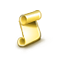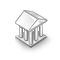Interesting script?
So post a link to it -
let others appraise it

You liked the script? Try it in the MetaTrader 5 terminalindicator for day trading 5 min chart - script for MetaTrader 4

Views:
1329
Rating:
Published:
2019.06.04 08:25
Updated:
2019.06.04 12:00

Here is the code if you directly wants to copy ,if you have downloaded the code from the above you need to set the chart in 5 min mode but in case you want to drag and drop , then copy the code below and go to your meta editor and then press ctrl+n or in mac command +n then choose Script then then  new and then just paste and save then restart the terminal and here you go.

```//+------------------------------------------------------------------+
//|                                                     RinkanKnight |
//|                                             https://www.mql5.com |
//+------------------------------------------------------------------+
#property version   "1.00"
#property strict
#property script_show_inputs

extern int     TakeProfit           = 10; //not necessary as this is a part of my EA this is here only
extern int     StopLoss             = 20;//not necessary as this is a part of my EA this is here only
extern double  lots =1 ;//not necessary as this is a part of my EA this is here only

void OnStart()
{

Alert("script started ");//to know the script started
static int  ticket = 0;//not necessary as this is a part of my EA this is here only
double TakeProfitLevel_buy = Bid + TakeProfit*Point;   //0.0001//not necessary as this is a part of my EA this is here only
double StopLossLevel_buy = Bid - StopLoss*Point;//not necessary as this is a part of my EA this is here only

double middlebb=iBands(Symbol(),5,20,2,0,PRICE_CLOSE,MODE_MAIN,0); //middle bb
double ma_ten = iMA(Symbol(),5,10,0,MODE_EMA,PRICE_CLOSE,0); // moving avg 10
double ma_fifty = iMA(Symbol(),5,50,0,MODE_EMA,PRICE_CLOSE,0); //moving avg 50
double sar = iSAR(Symbol(),5,0.02,0.2,0); // parabloic sar
double red = iStochastic(Symbol(),5,5,3,3,MODE_EMA,0,MODE_SIGNAL,0); // stochatic signal line
double blue = iStochastic(Symbol(),5,5,3,3,MODE_EMA,0,MODE_MAIN,0); // main stochatic line
double macd =iMACD(Symbol(),5,12,26,9,PRICE_CLOSE,MODE_MAIN,0);  // macd main
double rsi = iRSI(Symbol(),5,14,PRICE_CLOSE,0); //relative strength index

/*
Alert("the ckising price is ", Close
Alert("the bb  is ", middlebb );
Alert("the ma10 price is ", ma_ten );
Alert("the ma50e is ", ma_fifty );
Alert("the macd is ", macd );
Alert("the sar is ", sar );
Alert("the rsi is ", rsi );
Alert("the red signL is ", red );
Alert("the blue value is ", blue );

*/

if ( middlebb <Close && ma_ten < Close && ma_fifty < Close && sar < Close && blue > red && macd >0 && rsi >50 ) //checking condition for buy
{

}
else
if( middlebb > Close && ma_ten > Close && ma_fifty > Close && sar > Close && blue < red && macd < 0 && rsi < 50 )
{

}
else
{

}

}
```JohnBollinger_Ovrl

EA based on parameters of the BOOK of John Bollinger with Lot size calculator and position overlaping.Export Summary Grouped by Symbols (MT4)

The script exports summary of closed trades grouped by each currency pair to .csv fileMath Utils (MT4)

Handy functions for comparison and rounding of floating-point numbers (prices, lots and money).Adaptive Moving Average - AMA